# pylops.FirstDerivative¶

class pylops.FirstDerivative(N, dims=None, dir=0, sampling=1.0, edge=False, dtype='float64', kind='centered')[source]

First derivative.

Apply a first derivative using a three-point stencil finite-difference approximation.

Parameters: N : int Number of samples in model. dims : tuple, optional Number of samples for each dimension (None if only one dimension is available) dir : int, optional Direction along which the derivative is applied. sampling : float, optional Sampling step $$\Delta x$$. edge : bool, optional Use reduced order derivative at edges (True) or ignore them (False) for centered derivative dtype : str, optional Type of elements in input array. kind : str, optional Derivative kind (forward, centered, or backward).

Notes

The FirstDerivative operator applies a first derivative to any chosen direction of a multi-dimensional array using either a second-order centered stencil or first-order forward/backward stencils.

For simplicity, given a one dimensional array, the second-order centered first derivative is:

$y[i] = (0.5x[i+1] - 0.5x[i-1]) / \Delta x$

while the first-order forward stencil is:

$y[i] = (x[i+1] - x[i]) / \Delta x$

and the first-order backward stencil is:

$y[i] = (x[i] - x[i-1]) / \Delta x$
Attributes: shape : tuple Operator shape explicit : bool Operator contains a matrix that can be solved explicitly (True) or not (False)

Methods

 __init__(N[, dims, dir, sampling, edge, …]) Initialize this LinearOperator. adjoint() Hermitian adjoint. apply_columns(cols) Apply subset of columns of operator cond([uselobpcg]) Condition number of linear operator. conj() Complex conjugate operator div(y[, niter, densesolver]) Solve the linear problem $$\mathbf{y}=\mathbf{A}\mathbf{x}$$. dot(x) Matrix-matrix or matrix-vector multiplication. eigs([neigs, symmetric, niter, uselobpcg]) Most significant eigenvalues of linear operator. matmat(X) Matrix-matrix multiplication. matvec(x) Matrix-vector multiplication. rmatmat(X) Matrix-matrix multiplication. rmatvec(x) Adjoint matrix-vector multiplication. todense([backend]) Return dense matrix. toimag([forw, adj]) Imag operator toreal([forw, adj]) Real operator tosparse() Return sparse matrix. trace([neval, method, backend]) Trace of linear operator. transpose() Transpose this linear operator.

## Examples using pylops.FirstDerivative¶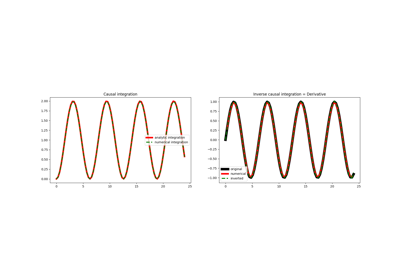Causal Integration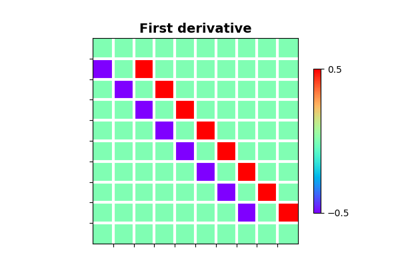Derivatives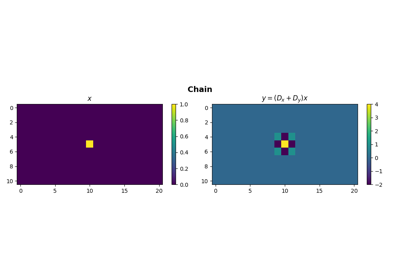Operators concatenation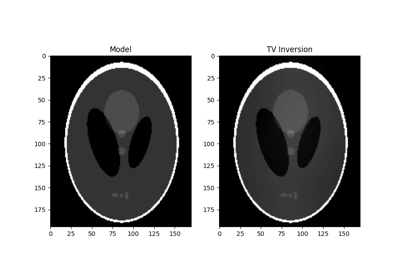Total Variation (TV) Regularization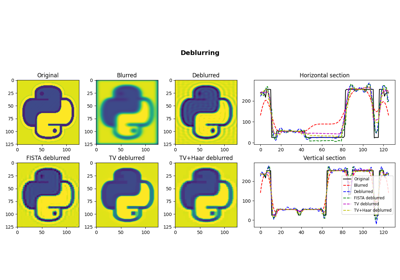05. Image deblurring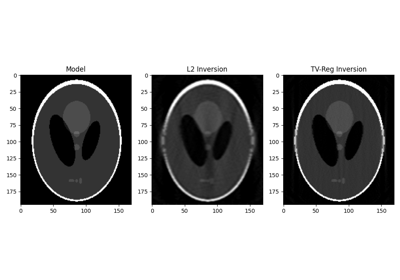16. CT Scan Imaging